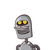# choose correct alternativeIf 49x – 57y = 172 and 57x – 49y = 252 then x – y = ……… ? (A) 80 (B) 4 (C) 10 (D) 8​

choose correct alternative
If 49x – 57y = 172 and 57x – 49y = 252 then x – y = ……… ?

(A) 80 (B) 4 (C) 10 (D) 8​

### 1 thought on “choose correct alternative<br />If 49x – 57y = 172 and 57x – 49y = 252 then x – y = ……… ? <br /><br />(A) 80 (B) 4 (C) 10 (D) 8​”

1.(C) 10

Step-by-step explanation:

57x – 49y = 252. Eqn 1

49x – 57y = 172 Eqn2

On subtracting Eqn 1 and 2 qe get

8x + 8y = 80 [divide by 8 on bothsides]

x + y =10